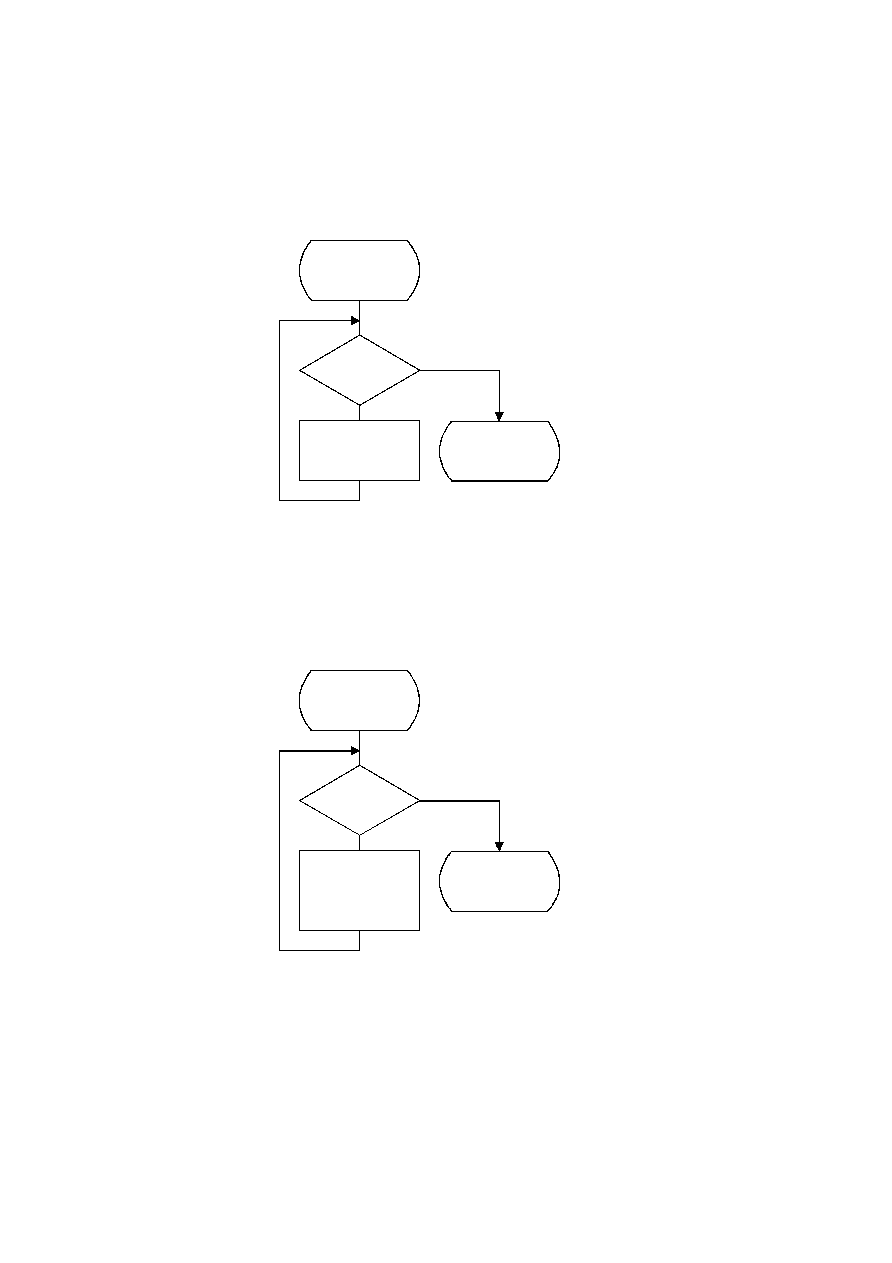ISO/IEC 10918-1 : 1993(E)
If a carry has not occurred, the output byte is tested to see if it is X'FF'. If so, the stack count ST is incremented, as the
output must be delayed until the carry-over is resolved. If not, the carry-over has been resolved, and any stacked X'FF'
bytes must then be placed in the entropy-coded segment. Note that a zero byte is stuffed following each X'FF'.
The procedures used by Byte_out are defined in Figures D.9 through D.11.
TISO1810-93/d047
Yes
No
Done
BP = BP + 1
B = 0
ST = ST ­ 1
Output_stacked_
zeros
ST = 0
?
Figure D.9 ­ Output_stacked_zeros procedure for encoder
Figure D.9 [D47], = 8.5 cm = 332.%
TISO1100-93/d048
Output_stacked_
X'FF's
Yes
No
Done
BP = BP + 1
B = X'FF'
BP = BP + 1
B = 0
ST = ST ­ 1
ST = 0
?
Figure D.10 ­ Output_stacked_X'FF's procedure for encoder
Figure D.10 [D48], = 8.5 cm = 332.%
CCITT Rec. T.81 (1992 E)
65